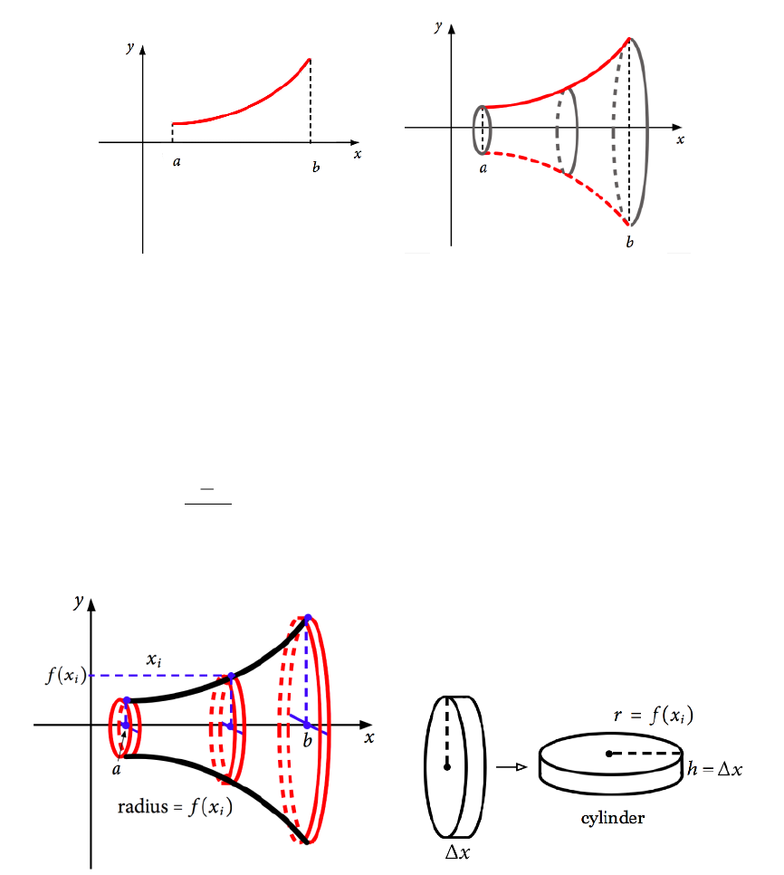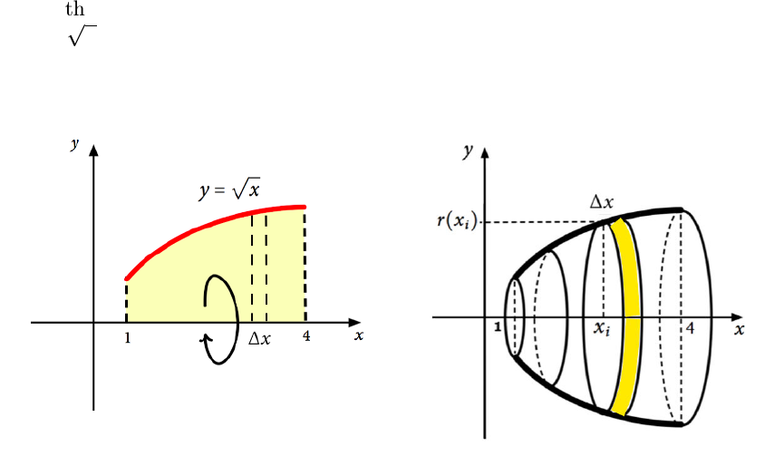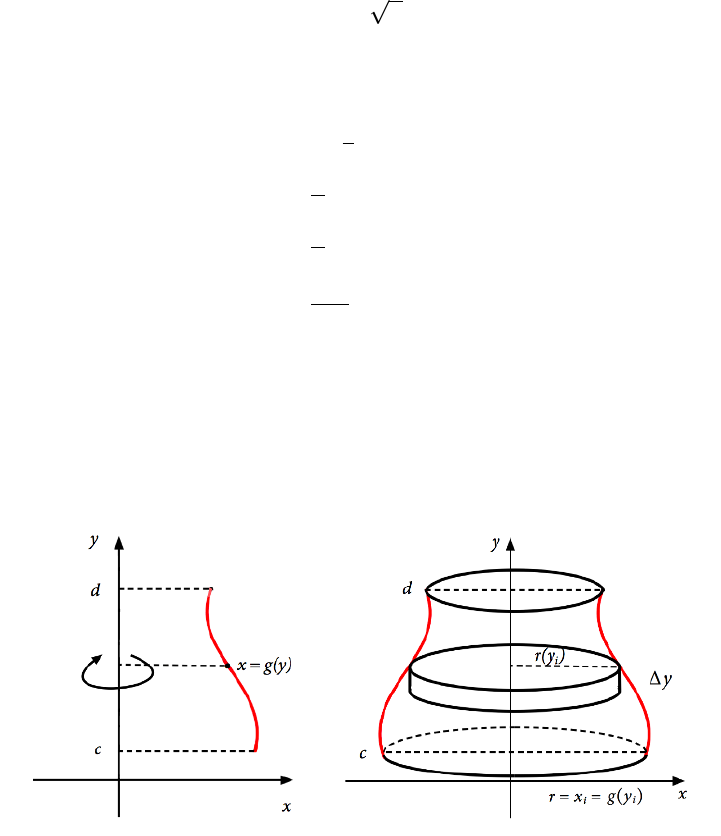Study Guides (400,000)
US (230,000)
NCSU (600)
MA (50)
MA 141 (40)
Final

# MA 141 Lecture Notes - Lecture 27: Riemann SumExam

Department
Mathematics
Course Code
MA 141
Professor
Kevin Flores
Study Guide
Final

This preview shows pages 1-3. to view the full 12 pages of the document.MA 141 Chapter 5
Section 5.2: Volumes of Solids of Revolution
In the previous sections, we used Riemann sums to approximate the area under a curve and
then deﬁned a deﬁnite integral as the exact area. We will use the same basic technique in
this section to ﬁnd the volume of certain solid shapes, called solids of revolution.
Def: Asolid of revolution is formed by revolving a planar region around a line in the
plane called its axis of revolution.
Method 1: Disks
When y=f(x)is a continuous function on [a, b]and Ais the planar region under the graph
of fand above the x-axis from x=ato x=b(area under the curve) and when we revolve
this region around the x-axis, we will use the disk method.
To ﬁnd the volume, we slice the solid into nvertical pieces, intersecting the x-axis at xi=
a+ixwhere x=ba
n. We approximate the volume of the slice using a cylinder of
height x.
1

Only pages 1-3 are available for preview. Some parts have been intentionally blurred.The volume of a cylinder is V=πr2h. Then the volume of this shape, using ndisks
(cylinders) to approximate, is
V
n
X
i=1
π(f(xi))2x.
As with our deﬁnition of area, the exact volume of this shape is obtained by taking the limit
as nincreases without bound.
V= lim
n→∞
n
X
i=1
π(f(xi))2x=Zb
a
π(f(x))2dx
To aid memory, we can label r(x) = f(x), where ris radius. Then
V=Zb
a
π(r(x))2dx.
Ex: Find the volume of the solid of revolution generated by revolving the region bounded
by f(x) = x,x= 1,x= 4 and the x-axis, about the x-axis.
Let’s look at the graph and the solid it generates:
( )== =
It should be easy enough to see that a vertical slice of this solid is roughly cylindrical, so the
disk method will work.

Only pages 1-3 are available for preview. Some parts have been intentionally blurred.V=Zb
a
π(r(x))2dx
=Z4
1
π(x)2dx
=Z4
1
πx dx
=π·1
2x2
4
1
=π
2(4212)
=π
2(15)
=15π
2
23.6
Since the units are not speciﬁed here, we can simply say 23.6 cubic units of volume.
Notice that we could also revolve a function x=g(y)about the y-axis to generate a solid of
revolution.
Here, the volume is given by
V=Zd
c
π(g(y))2dy =Zd
c
π(r(y))2dy.